# Civil Engineering - Surveying

### Exercise :: Surveying - Section 1

11.

One of the Lehmann's rules of plane tabling, is

 A. location of the instrument station is always distant from each of the three rays from the known points in proportion to their distances B. when looking in the direction of each of the given points, the instrument station will be on the right side of one and left side of the other ray C. when the instrument station is outside the circumscribing circle its location is always on the opposite side of the ray to the most distant point as the inter-section of the other two rays D. none of these.

Explanation:

No answer description available for this question. Let us discuss.

12.

If f1 and f2 are the distances from the optical centre of a convex lens of focal length f to conjugate two points P1 and P2 respectively, the following relationship holds good

 A. f = f1 + f2 B. f =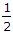(f1 + f2) C.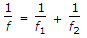D. none of these.

Explanation:

No answer description available for this question. Let us discuss.

13.

The accuracy of measurement in chain surveying, does not depend upon

 A. length of the offset B. scale of the plotting C. importance of the features D. general layout of the chain lines.

Explanation:

No answer description available for this question. Let us discuss.

14.

If arithmetic sum of latitudes of a closed traverse is ∑Lat and closing error in latitude is dx, the correction for a side whose latitude is l, as given by Transit Rule, is

 A.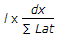B.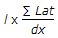C.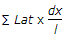D. none of these.

Explanation:

No answer description available for this question. Let us discuss.

15.

Closed contours of decreasing values towards their centre, represent

 A. a hill B. a depression C. a saddle or pass D. a river bed.